# Search by Topic

#### Resources tagged with Making and proving conjectures similar to Integral Sandwich:

Filter by: Content type:
Age range:
Challenge level:

### There are 45 results

Broad Topics > Using, Applying and Reasoning about Mathematics > Making and proving conjectures### Integral Sandwich

##### Age 16 to 18 Challenge Level:

Generalise this inequality involving integrals.### Fibonacci Factors

##### Age 16 to 18 Challenge Level:

For which values of n is the Fibonacci number fn even? Which Fibonnaci numbers are divisible by 3?### Multiplication Arithmagons

##### Age 14 to 16 Challenge Level:

Can you find the values at the vertices when you know the values on the edges of these multiplication arithmagons?### Loopy

##### Age 14 to 16 Challenge Level:

Investigate sequences given by $a_n = \frac{1+a_{n-1}}{a_{n-2}}$ for different choices of the first two terms. Make a conjecture about the behaviour of these sequences. Can you prove your conjecture?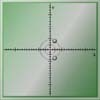### Conjugate Tracker

##### Age 16 to 18 Challenge Level:

Make a conjecture about the curved track taken by the complex roots of a quadratic equation and use complex conjugates to prove your conjecture.### Plus or Minus

##### Age 16 to 18 Challenge Level:

Make and prove a conjecture about the value of the product of the Fibonacci numbers $F_{n+1}F_{n-1}$.### Cyclic Triangles

##### Age 16 to 18 Challenge Level:

Make and prove a conjecture about the cyclic quadrilateral inscribed in a circle of radius r that has the maximum perimeter and the maximum area.### Discrete Trends

##### Age 16 to 18 Challenge Level:

Find the maximum value of n to the power 1/n and prove that it is a maximum.### An Introduction to Magic Squares

##### Age 7 to 16### Summats Clear

##### Age 16 to 18 Challenge Level:

Find the sum, f(n), of the first n terms of the sequence: 0, 1, 1, 2, 2, 3, 3........p, p, p +1, p + 1,..... Prove that f(a + b) - f(a - b) = ab.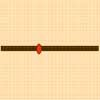### Least of All

##### Age 16 to 18 Challenge Level:

A point moves on a line segment. A function depends on the position of the point. Where do you expect the point to be for a minimum of this function to occur.### 2^n -n Numbers

##### Age 16 to 18

Yatir from Israel wrote this article on numbers that can be written as $2^n-n$ where n is a positive integer.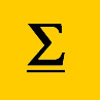### The Kth Sum of N Numbers

##### Age 16 to 18

Yatir from Israel describes his method for summing a series of triangle numbers.### Why Stop at Three by One

##### Age 16 to 18

Beautiful mathematics. Two 18 year old students gave eight different proofs of one result then generalised it from the 3 by 1 case to the n by 1 case and proved the general result.### Binary Squares

##### Age 16 to 18 Challenge Level:

If a number N is expressed in binary by using only 'ones,' what can you say about its square (in binary)?### Poly Fibs

##### Age 16 to 18 Challenge Level:

A sequence of polynomials starts 0, 1 and each poly is given by combining the two polys in the sequence just before it. Investigate and prove results about the roots of the polys.### Fibonacci Fashion

##### Age 16 to 18 Challenge Level:

What have Fibonacci numbers to do with solutions of the quadratic equation x^2 - x - 1 = 0 ?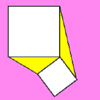### Trig Rules OK

##### Age 16 to 18 Challenge Level:

Change the squares in this diagram and spot the property that stays the same for the triangles. Explain...### What's Possible?

##### Age 14 to 16 Challenge Level:

Many numbers can be expressed as the difference of two perfect squares. What do you notice about the numbers you CANNOT make?### OK! Now Prove It

##### Age 16 to 18 Challenge Level:

Make a conjecture about the sum of the squares of the odd positive integers. Can you prove it?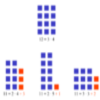### Prime Sequences

##### Age 16 to 18 Challenge Level:

This group tasks allows you to search for arithmetic progressions in the prime numbers. How many of the challenges will you discover for yourself?##### Age 14 to 16 Challenge Level:

The points P, Q, R and S are the midpoints of the edges of a non-convex quadrilateral.What do you notice about the quadrilateral PQRS and its area?##### Age 14 to 16 Challenge Level:### Close to Triangular

##### Age 14 to 16 Challenge Level:

Drawing a triangle is not always as easy as you might think!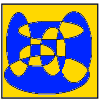### Painting by Numbers

##### Age 16 to 18 Challenge Level:

How many different colours of paint would be needed to paint these pictures by numbers?### Pythagorean Fibs

##### Age 16 to 18 Challenge Level:

What have Fibonacci numbers got to do with Pythagorean triples?### The Clue Is in the Question

##### Age 16 to 18 Challenge Level:

Starting with one of the mini-challenges, how many of the other mini-challenges will you invent for yourself?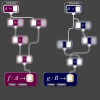### Steve's Mapping

##### Age 16 to 18 Challenge Level:

Steve has created two mappings. Can you figure out what they do? What questions do they prompt you to ask?### Multiplication Square

##### Age 14 to 16 Challenge Level:

Pick a square within a multiplication square and add the numbers on each diagonal. What do you notice?### Fixing It

##### Age 16 to 18 Challenge Level:

A and B are two fixed points on a circle and RS is a variable diamater. What is the locus of the intersection P of AR and BS?### Triangles Within Triangles

##### Age 14 to 16 Challenge Level:

Can you find a rule which connects consecutive triangular numbers?### Polite Numbers

##### Age 16 to 18 Challenge Level:

A polite number can be written as the sum of two or more consecutive positive integers. Find the consecutive sums giving the polite numbers 544 and 424. What characterizes impolite numbers?### DOTS Division

##### Age 14 to 16 Challenge Level:

Take any pair of two digit numbers x=ab and y=cd where, without loss of generality, ab > cd . Form two 4 digit numbers r=abcd and s=cdab and calculate: {r^2 - s^2} /{x^2 - y^2}.### Sixty-seven Squared

##### Age 16 to 18 Challenge Level:

Evaluate these powers of 67. What do you notice? Can you convince someone what the answer would be to (a million sixes followed by a 7) squared?### Janine's Conjecture

##### Age 14 to 16 Challenge Level:

Janine noticed, while studying some cube numbers, that if you take three consecutive whole numbers and multiply them together and then add the middle number of the three, you get the middle number. . . .### To Prove or Not to Prove

##### Age 14 to 18

A serious but easily readable discussion of proof in mathematics with some amusing stories and some interesting examples.### On the Importance of Pedantry

##### Age 16 to 18

A introduction to how patterns can be deceiving, and what is and is not a proof.### Triangles Within Pentagons

##### Age 14 to 16 Challenge Level:

Show that all pentagonal numbers are one third of a triangular number.### Triangles Within Squares

##### Age 14 to 16 Challenge Level:

Can you find a rule which relates triangular numbers to square numbers?### Problem Solving, Using and Applying and Functional Mathematics

##### Age 5 to 18 Challenge Level:

Problem solving is at the heart of the NRICH site. All the problems give learners opportunities to learn, develop or use mathematical concepts and skills. Read here for more information.### Polycircles

##### Age 14 to 16 Challenge Level:

Show that for any triangle it is always possible to construct 3 touching circles with centres at the vertices. Is it possible to construct touching circles centred at the vertices of any polygon?### How Old Am I?

##### Age 14 to 16 Challenge Level:

In 15 years' time my age will be the square of my age 15 years ago. Can you work out my age, and when I had other special birthdays?### A Little Light Thinking

##### Age 14 to 16 Challenge Level:

Here is a machine with four coloured lights. Can you make two lights switch on at once? Three lights? All four lights?### Curvy Areas

##### Age 14 to 16 Challenge Level:

Have a go at creating these images based on circles. What do you notice about the areas of the different sections?### Few and Far Between?

##### Age 14 to 18 Challenge Level:

Can you find some Pythagorean Triples where the two smaller numbers differ by 1?